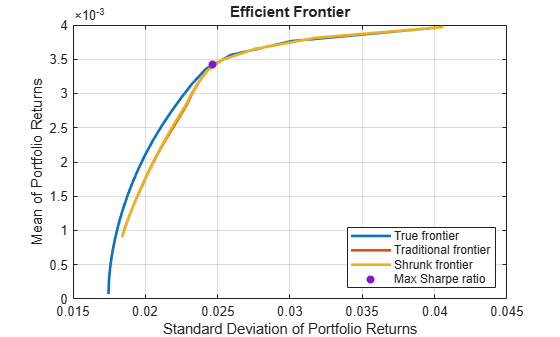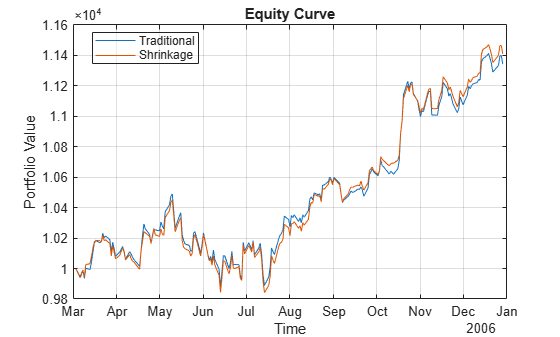# covarianceShrinkage

Estimate covariance matrix using shrinkage estimators

Since R2023a

## Syntax

``SigmaHat = covarianceShrinkage(AssetReturns)``

## Description

example

````SigmaHat = covarianceShrinkage(AssetReturns)` returns a covariance estimate using linear shrinkage to reduce the mean squared error (MSE).`covarianceShrinkage` computes an estimate of the covariance matrix from a sample of asset returns using the multiple of the identity shrinkage estimation method. For more information, see Covariance Shrinkage and covarianceShrinkage Algorithm.In addition, you can use `covarianceDenoising` to compute an estimate of covariance matrix using denoising. For information on which covariance estimation method to choosesee Comparison of Methods for Covariance Estimation.```

## Examples

collapse all

This example shows how to use `covarianceShrinkage` to compute covariance estimations that take into account the noise in the sample. In mean-variance portfolio optimization, a noisy estimate of the covariance estimate results in unstable solutions that cause high turnover and transaction costs. Ideally, to decrease the estimation error, it is desirable to increase the sample size. Yet, there are cases where this is not possible. In extreme cases in which the number of assets is larger than the number of observations, the traditional covariance matrix results in a singular matrix. Working with a nearly singular or an ill-conditioned covariance matrix magnifies the impact of estimation errors.

Compute a portfolio efficient frontier using different covariance estimates with the same sample data.

```% Load portfolio data with 225 assets load port5.mat covariance = corr2cov(stdDev_return,Correlation); % Generate a sample with 200 observations rng('default') nScen = 200; retSeries = portsim(mean_return',covariance,nScen);```

Compute the traditional and shrunk covariance estimates. Use `covarianceShrinkage` to reduce the effect of noise in the covariance approximation.

```Sigma = cov(retSeries); shrunkSigma = covarianceShrinkage(retSeries);```

Compute the condition number of both covariance estimates. The shrunken covariance (`shrunkSigma)` has a lower condition number than the traditional covariance estimate `Sigma`.

```conditionNum = [cond(Sigma); cond(shrunkSigma)]; condNumT = table(conditionNum,'RowNames',{'Sigma','SigmaHat'})```
```condNumT=2×1 table conditionNum ____________ Sigma 6.0141e+18 SigmaHat 5274.8 ```

Use `Portfolio` to construct `Portfolio` objects that use the different `AssetCovar` values. Then use `setDefaultConstraints` to set the portfolio constraints with nonnegative weights that sum to 1 for the three portfolios: the true mean with the true covariance, the traditional covariance estimate, and the shrunk estimate.

```% Create a Portfolio object with the true parameters p = Portfolio(AssetMean=mean_return,AssetCovar=covariance); p = setDefaultConstraints(p); % Create a Portfolio object with true mean and traditional covariance estimate pTraditional = Portfolio(AssetMean=mean_return,AssetCovar=Sigma); pTraditional = setDefaultConstraints(pTraditional); % Create a Portfolio object with true mean and shrunk covariance pShrunk = Portfolio(AssetMean=mean_return,AssetCovar=shrunkSigma); pShrunk = setDefaultConstraints(pShrunk);```

Use `estimateFrontier` to estimate the efficient frontier for each of the `Portfolio` objects.

```% Number of portfolios on the efficient frontier nPort = 20; % True efficient portfolios w = estimateFrontier(p,nPort); % Traditional covariance efficient portfolios wTraditional = estimateFrontier(pTraditional,nPort); % Denoised covariance efficient portfolios wShrunk = estimateFrontier(pShrunk,nPort);```

Use `plotFrontier` to plot the frontier obtained from the different weights using the true parameter values.

```figure plotFrontier(p,w) hold on plotFrontier(p,wTraditional) plotFrontier(p,wShrunk) plotFrontier(p,estimateMaxSharpeRatio(p)) legend('True frontier','Traditional frontier','Shrunk frontier', ..., 'Max Sharpe ratio',Location='southeast'); hold off```In this example, the efficient frontiers obtained using the traditional covariance estimate and the shrunk estimate are close to each other. This means that both methods achieve similar risk and returns out-of-sample. Where the difference between these methods is more noticeable is for the portfolios to the left of the maximum Sharpe ratio portfolio. For those portfolios, the allocation computed using shrinkage has better returns out-of-sample.

This example compares a minimum variance investment strategy using the traditional covariance estimate with a minimum variance strategy using covariance shrinkage.

```% Read a table of daily adjusted close prices for 2006 DJIA stocks. T = readtable('dowPortfolio.xlsx'); % Convert the table to a timetable. pricesTT = table2timetable(T,'RowTimes','Dates'); numAssets = size(pricesTT.Variables, 2);```

Use the first 42 days of the `dowPortfolio.xlsx` data set to initialize the backtest strategies. The backtest is then run over the remaining data.

`warmupPeriod = 42;`

Compute the initial weights. Use the `traditionalStrat` and `shrunkStrat` functions in Local Functions to compute the weights.

```% Specify no current weights (100% cash position). w0 = zeros(1,numAssets); % Specify warm-up partition of data set timetable. warmupTT = pricesTT(1:warmupPeriod,:); % Compute the initial portfolio weights for each strategy. traditional_initial = traditionalStrat(w0,warmupTT); shrunk_initial = shrunkStrat(w0,warmupTT);```

Create traditional and shrinkage backtest strategy objects using `backtestStrategy`.

```% Rebalance approximately every month. rebalFreq = 21; % Set the rolling lookback window to be at least 2 months and at % most 6 months. lookback = [42 126]; % Use a fixed transaction cost (buy and sell costs are both 0.5% % of amount traded). transactionsFixed = 0.005; % Specify the strategy objects. strat1 = backtestStrategy('Traditional', @traditionalStrat, ... RebalanceFrequency=rebalFreq, ... LookbackWindow=lookback, ... TransactionCosts=transactionsFixed, ... InitialWeights=traditional_initial); strat2 = backtestStrategy('Shrinkage', @shrunkStrat, ... RebalanceFrequency=rebalFreq, ... LookbackWindow=lookback, ... TransactionCosts=transactionsFixed, ... InitialWeights=shrunk_initial); % Aggregate the two strategy objects into an array. strategies = [strat1, strat2];```

Create a `backtestEngine` object then use `runBacktest` to run the backtest.

```% Create the backtesting engine object. backtester = backtestEngine(strategies); % Run the backtest. backtester = runBacktest(backtester,pricesTT,'Start',warmupPeriod); % Generate summary table of the performance of the strategies. summary(backtester)```
```ans=9×2 table Traditional Shrinkage ___________ __________ TotalReturn 0.13431 0.14101 SharpeRatio 0.10807 0.11565 Volatility 0.0057472 0.0056077 AverageTurnover 0.0098378 0.0077384 MaxTurnover 0.36237 0.31835 AverageReturn 0.0006196 0.00064699 MaxDrawdown 0.058469 0.058133 AverageBuyCost 0.51636 0.40533 AverageSellCost 0.51636 0.40533 ```

Use `equityCurve` to plot the equity curve to compare the performance of both strategies.

`equityCurve(backtester)`The maximum and average turnover are decreased using covariance shrinkage. Also, the covariance shrinkage strategy results in a decrease of buy and sell costs. In this example, not only is the turnover decreased, but also the volatility and the maximum drawdown are decreased. Therefore, in this example the shrunk covariance produces more robust weights.

Local Functions

```function new_weights = traditionalStrat(~, pricesTT) % Function for minimum variance portfolio using traditional covariance estimate. % Compute the returns from the prices timetable. assetReturns = tick2ret(pricesTT); mu = mean(assetReturns.Variables); Sigma = cov(assetReturns.Variables,"omitrows"); % Create the portfolio problem. p = Portfolio(AssetMean=mu,AssetCovar=Sigma); % Specify long-only fully invested contraints. p = setDefaultConstraints(p); % Compute the minimum variance portfolio. new_weights = estimateFrontierLimits(p,'min'); end function new_weights = shrunkStrat(~, pricesTT) % Function for minimum variance portfolio using covariance shrinkage. % Compute the returns from the prices timetable. assetReturns = tick2ret(pricesTT); mu = mean(assetReturns.Variables); Sigma = covarianceShrinkage(assetReturns.Variables); % Create the portfolio problem. p = Portfolio(AssetMean=mu,AssetCovar=Sigma); % Specify long-only fully invested contraints. p = setDefaultConstraints(p); % Compute the minimum variance portfolio. new_weights = estimateFrontierLimits(p,'min'); end```

## Input Arguments

collapse all

Asset returns, specified as a `NumObservations`-by-`NumAssets` matrix, table, or timetable.

Note

All `NumObservations` with one or more `NaN` values are removed before computing the covariance estimate.

Data Types: `double` | `table` | `timetable`

## Output Arguments

collapse all

Covariance estimate, returned as a `NumAssets`-by-`NumAssets` matrix.

collapse all

### Covariance Shrinkage

Shrinkage estimators for covariance shrinkage are used to reduce the effect of noise in the covariance approximation.

The goal of covariance shrinkage is to pull all eigenvalues of the traditional covariance matrix towards a target.

## Algorithms

The `covarianceShrikage` function applies a linear shrinkage method that shrinks the traditional covariance estimate to a multiple of the identity matrix.

`$\stackrel{^}{\Sigma }=\left(1-\alpha \right)\Sigma +\alpha \left(\tau I\right)$`

Here, Σ is the standard covariance estimate, τ is the average sample variance, and $\alpha \in \left[0,1\right]$ is the intensity parameter computed using

`$\alpha =\frac{\frac{1}{N}\sum _{i=1}^{N}\text{trace(}{\left[{z}_{i}{z}_{i}^{T}-\Sigma \right]}^{2}\right)}{\text{trace(}{\left[\Sigma \text{-}\tau \right]}^{2}\right)}$`

where zi is the i th row of the centered sample matrix Z and N is the sample size.

 Ledoit, O. and Wollf, M. "A Well-Conditioned Estimator for Large-Dimensional Covariance Matrices." Journal of Multivariate Analysis. vol. 88, no. 2, 365–411, 2004.